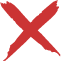# Weighted Average Calculator

This weighted average calculator calculates your average of all your courses. The weighted average simply takes into account how much each course is worth when calculating the average. This calculator can also be used to calculate any weighted average, not just courses.

12345